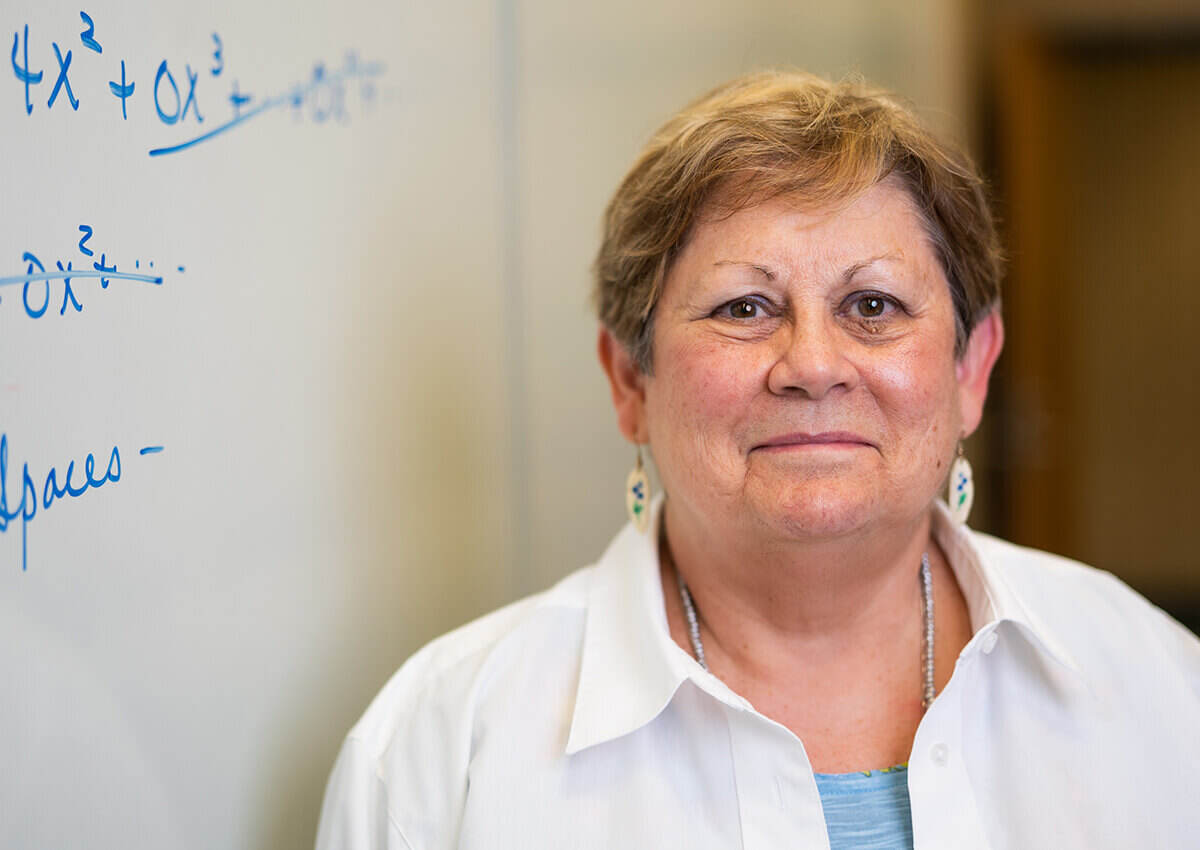### Philippians 1:6# Lois Knouse, M.M.

Phone: 903.233.3338
Email: LoisKnouse@letu.edu

• # Associate Professor Emeritus, Mathematics

### Education

M.M., University of Tennessee, 1969
B.A., LeTourneau University, 1967

### Courses Taught

• MATH 1003 – Introductory Algebra
• MATH 1123 – Intermediate Algebra
• MATH 1152 - Trigonometry
• MATH 1203 – College Algebra
• MATH 1303 – Precalculus
• MATH 1423 – Elementary Statistics
• MATH 1503 – Calculus with Business Applications
• MATH 1603 – Technical Calculus I
• MATH 1613 – Technical Calculus II
• MATH 1903 – Calculus I
• MATH 2003 – Discrete Mathematics
• MATH 2013 – Calculus II
• MATH 2023 – Calculus III
• MATH 2203 – Differential Equations
• MATH 2303 – Linear Algebra
• MATH 2503 – Logic, Sets, and Proofs
• MATH 3703 – Abstract Algebra
• MATH 4403 – Numerical Analysis

### Online Courses Written

• MATH 1303 – Precalculus – including 64 videos
• MATH 1903 – Calculus I – including 84 videos